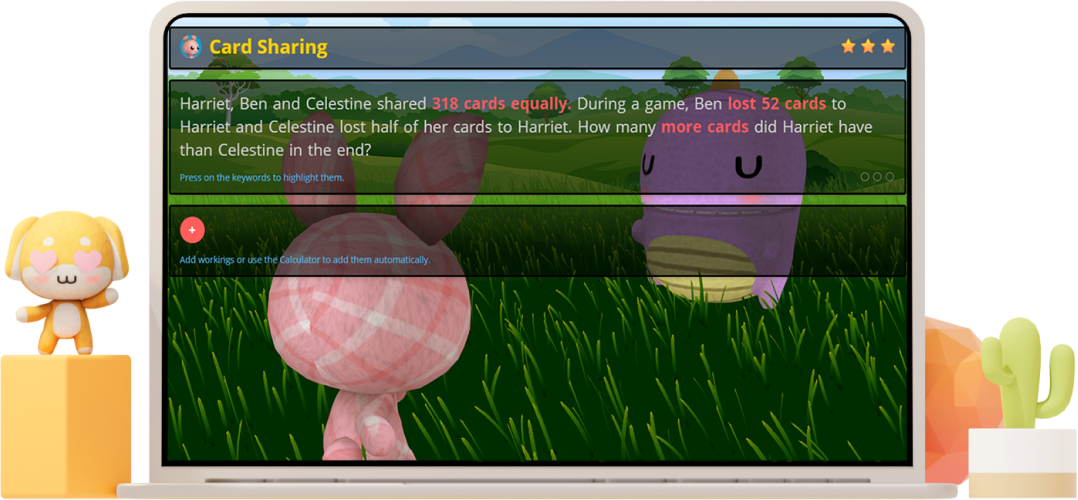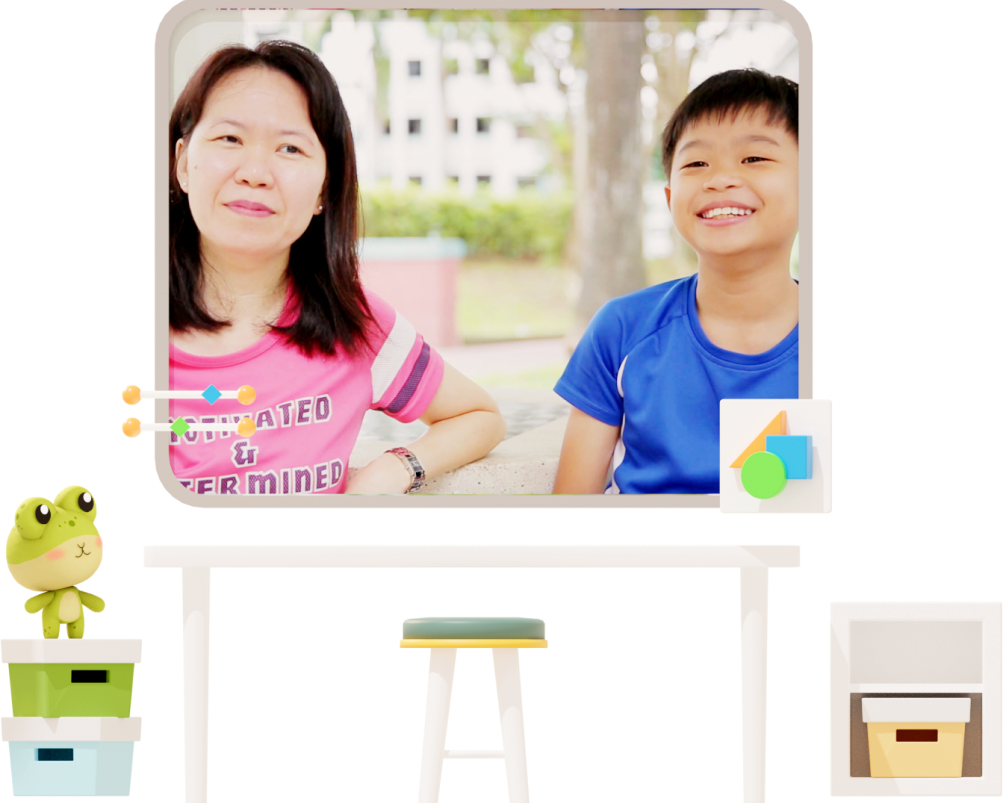Math problem sums

## 11 Math problem sums every AL1 student knows

Math problem sums (or Math word problems) has always been a challenge to many primary school kids and their parents. If you are one of them who’s having trouble solving these primary school math problems, we’re here to help!

In this Practicle Math problem sums guide, we are going to identify 11 types of word problem sums that you’ll often see in your primary school math homework & school examinations. Besides that, we’ll also be looking at some of the best problem-solving methods to help get you to the solution quick.

Once you get the hang of identifying the math concepts you are being tested in each question, solving problem sums and finding the right answer is going to be easy peasy! Ready to start?

## What are problem sums?

You can think of problem sums that the Math word problems that you see mostly in your Mathematics Paper 2. These word problems are usually quite lengthy and consist of a few sentences that describes a situation (the problem) where you need to apply some kind of Math concepts to calculate and solve.

At the primary school level, we can classify these Math concepts that you need to know into 11 types. Let’s see what kind of Math problem sums question types you can expect to see in middle primary and upper primary.

### 1. Remainder concept

Math questions that use the Remainder Concept are often problem sums that contain the word “remainder” or involves some kind of left-over. Most of these remainder concept problem sums either deal with whole numbers or fractions.

Here’s an example question in Primary 4 that uses the Remainder Concept:

Wendy spent 4/7 of her money on a pair of shoes and 2/3 of the remainder on a pair of earrings. Given that she had \$10 left, how much did Wendy have at first?

### What’s the best way to solve these maths sums easily?

You can solve such Math problem sums by using the model drawing method (with part-whole models) or the branching method.

### 2. Proportion concept

The Proportion concept forms the basis of most Math questions. However, they can be hard to solve for many students.

You will see that these problem sums will provide your child with the total quantity of the items, the proportion of one item to the other and a common characteristic between the items.

Here’s a Primary 3 Math question example with this concept:

Gary spent \$1760 on some tables and chairs. He bought 20 more chairs than tables. If each table cost \$77 and each chair cost \$33, find the number of chairs he bought.

### What’s the best way to solve these maths sums easily?

To solve them, simply apply the grouping method and you’ll be well on your way to getting the right answer.

### 3. Simultaneous concept

The Proportion concept forms the basis of most Math questions. However, they can be hard to solve for many students.

Simultaneous concept problem sums can vary in terms of difficulty. Although the concept is similar to simultaneous equations in Algebra in secondary school, the numbers that are used in such Math questions at the primary school level are usually more friendly.

Here’s an example question in Primary 4 that uses this Math concept:

There are 42 children in a canteen. 2/5 of the boys and 1/2 of the girls like to eat fried chicken wings. There are 19 children who like to eat fried chicken wings. Find the number of boys in the canteen.

### What’s the best way to solve these maths sums easily?

In order to solve problem sums that compares different quantities of the same items, you can work with the relationship between the numbers or use the model method.

### Master problem sums in a matter of minutes, not hours.### 4. Pattern concept

Instead of problem sums, these Math questions can be thought of as puzzles. Depending on how good your spatial and temporal skills are, Math questions that uses the Pattern Concept can be the one of the easiest or most challenging ones.

Here’s an example question on patterns from Primary 3:

The pattern below is made up by arranging some tables and chairs. Study the pattern carefully and answer the questions below. How many chairs are there in Pattern 48?

### What’s the best way to solve these maths sums easily?

The key to solving these questions is to link what you see in the puzzle to familiar numbers. There is no fixed way to solve such questions and there are usually more than one way of solving them.

### 5. Gap and difference concept

The last type of Math problem sum question that we are going to look at involves the infamous Gap and Difference Concept (also known as the Excess and Shortage Concept).

These questions usually give us two scenarios that compares the quantities of two items and you’ll have too much of one item or too little of the item.

Here’s an example of such a Math question from Practicle:

Aunt May wanted to give some children a red packet each for Chinese New Year. If she gives each child \$5, she will have \$24 left. If she gives each child \$8, she will have \$6 left. How many children were there?

### What’s the best way to solve these maths sums easily?

To solve these Gap and Difference questions, you can use the model method, the units method or purely through simple arithmetic.

### 6. Equal concept

Math problem sums that uses the Equal Concept in P5 and P6 compare two fractions (sometimes expressed in percentages) of different items that represent equal amounts.

Here’s an example question that we’ve shared on our Practicle Math channel:

Thor and Hulk punched each other multiple times in the Contest of Champions battle. 3/4 of Thor’s punches were equal to 2/3 of Hulk’s punches. Both of them punched a total of 408 times. How many times did Thor punch the Hulk?

### What’s the best way to solve these maths sums easily?

Use either model drawing (comparison models) or try to make the numerators of both fraction the same.

### 7. Repeated identity concept

Like its name, the Repeated Identity Concept appears in word problems where there exist 2 or 3 items with a common quantity.

Here’s an example question that you might see in P5:

Doodles has 2/3 as many shiny cards as Ash. Ash has 1/5 as many shiny cards as Yugi. If the three of them have a total of 120 cards, how many shiny cards does Doodles have?

### What’s the best way to solve these maths sums easily?

To solve these Math questions, we’ll first need to identify the common quantity that is given in that particular problem sum. Once we spot that, we can then solve it using the units method.

Now, let’s look at 4 different transfer type problem sums.

Understanding the main idea behind each Math concept is key to solving these problem sums.

### 8. External transfer (Unchanged quantity) concept

Such problem sums have two items involved and an event happening that results in a change in the quantity of one of the items while the other quantity remains the same.

Here’s a P6 Math example question that uses this Math concept:

There are 80 children at a swim club. 30% of them were boys. After a few boys left the club, the percentage of boys dropped to 20%. How many boys left the club?

### What’s the best way to solve these maths sums easily?

You can solve these questions either by using the Singapore bar model method or the units method if the number is not as friendly.

### 9. Internal transfer (Unchanged total) concept

Similar to what we discussed earlier on, these type of Math problem sums questions also involves two items and an event happening.

However, because the event usually refers to a transfer of a certain quantity of one item to the other, the quantity of each item changes. The only thing that remains constant is the total quantity of the two items.

Here’s an example question that you may see in Primary 5:

The ratio of Tom’s allowance to Jerry’s allowance is 7:11. If Jerry gives Tom \$84, they will have the same amount of money. How much do they have altogether?

### What’s the best way to solve these maths sums easily?

To find the answer, we can either draw the model to solve this question or use the units method.

### 10. External transfer (Same difference) concept

Next, let’s look at Math problem sums that deals with the External Transfer Concept, also known as the Constant Difference Concept.

In these problem sums, the same quantity of each item will be added or subtracted from the two existing items that we have, Therefore, we’ll know for sure that the difference between the items will stay the same.

Here’s a Primary 6 example question:

At first, Jay had \$1144 while Samuel had \$526. After they each spend an equal amount of money on some toys, Jay then had 4 times as much money as Samuel. How much did each boy spend?

### What’s the best way to solve these maths sums easily?

Once again, the Bar Model Method or the Units Method will come in handy when we are working out the answer.

### 11. External transfer (Changed quantity) concept

The last type of transfer problem sums deals with the External transfer with changed quantity concept. This is one of the most difficult Math problem sums to master.

In these Math questions, the quantity of the two items given at the start and the end are different and it may be hard for children to visualise what is going on with the Model Method. Hence, solving them successfully usually requires using the Units and Parts method, which is a simplified form of Algebra using two variables.

Here’s an example question for Primary 6 PSLE:

Snow had 40% as many apples as Sleepy at first. Snow bought another 8 apples and Sleepy ate 5 apples. Then, Snow had 80% as many apples as Sleepy. Find the number of apples Snow had at first.

### What’s the best way to solve these maths sums easily?

Once again, the Bar Model Method or the Units Method will come in handy when we are working out the answer.

### Feeling more confident about Math problem sums now?

We hope that this Practicle Problem Sums Guide was able to paint a clearer picture of the kind of Math problem sum questions that your kid is facing in primary school.

If you want to learn how to solve such Math problem sums step by step and master every Math concept you need to know, see how Practicle’s fun and adaptive Math practice system can help.Real story

## No more problems with problem sums

From 68 to 97 marks - Cayden's grades dropped when he started P5 so his mum decided to try Practicle!